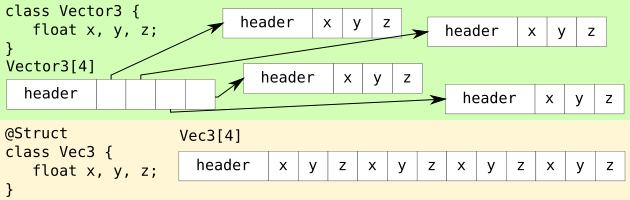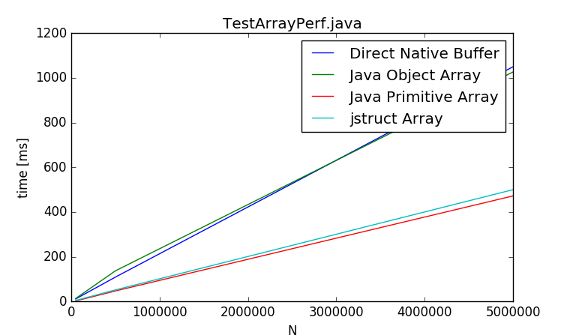## Project JUnionDelivers struct types to Java programming language.

When creating arrays of int, we have two main options:

``````int[] intArray = new int;
Integer[] intBoxedArray = new Integer;
``````

How many bytes do `intArray, intBoxedArray` take to store 1000 ints?

`intArray` 4016 bytes `4*1000 + ~16(around 16 bytes for array header)`
`intBoxedArray` 20016 bytes `(4 + ~12 + ~4)*1000 + ~16` (exact number depends on VM)

That is almost 5x more!
Well, this teaches us to prefer primitive arrays over their boxed versions.
So what is this project about?

Consider

``````class Point { float x,y;}
Point[] arr = new Point;
``````

`arr` takes 14016 bytes
The data consits of 500 points, 2 floats each, thus 4000 bytes should be enough.
If Point was a struct, `arr` would take ~4000 bytes.

Wouldn’t it be nice to be able to create struct types in Java that code like class and work like structs?

With JUnion you can do just that by marking a class with @Struct annotation!

Create struct Vec3:

``````@Struct
public class Vec3 {
public float x,y,z;
}
``````

Afterwards you can use it as:

``````//Create a new struct array
Vec3[] arr = new Vec3;
arr.x = 10;
Vec3 v = arr;
...
//
ByteBuffer a = ByteBuffer.allocateDirect(10*Mem.sizeOf(Vec3.class))
.order(ByteOrder.nativeOrder());
//Modify Direct Native Bytebuffer as it were a struct
Vec3[] arr = Mem.wrap(a);
arr.x = 10;
...
``````